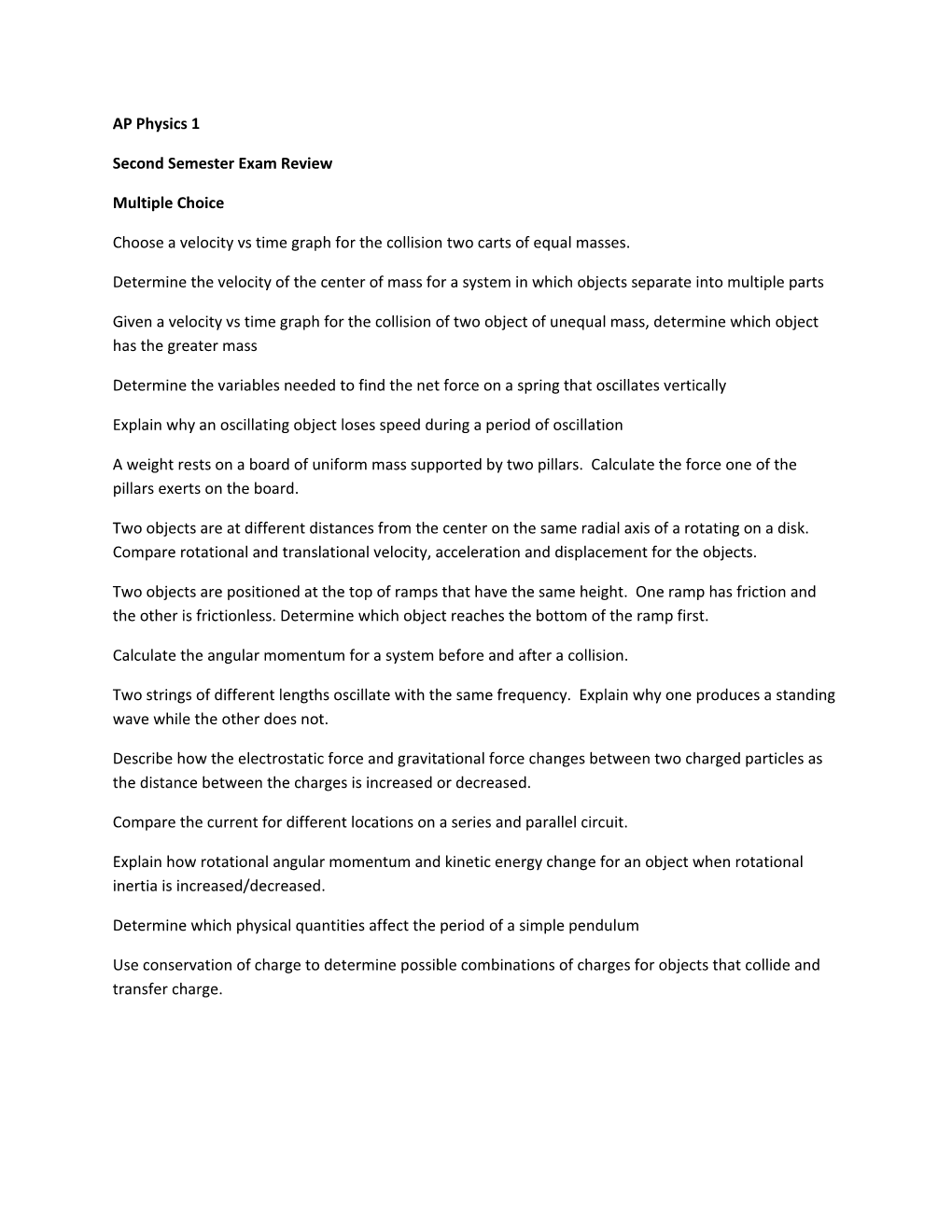# Second Semester Exam ReviewAP Physics 1

Second Semester Exam Review

Multiple Choice

Choose a velocity vs time graph for the collision two carts of equal masses.

Determine the velocity of the center of mass for a system in which objects separate into multiple parts

Given a velocity vs time graph for the collision of two object of unequal mass, determine which object has the greater mass

Determine the variables needed to find the net force on a spring that oscillates vertically

Explain why an oscillating object loses speed during a period of oscillation

A weight rests on a board of uniform mass supported by two pillars. Calculate the force one of the pillars exerts on the board.

Two objects are at different distances from the center on the same radial axis of a rotating on a disk. Compare rotational and translational velocity, acceleration and displacement for the objects.

Two objects are positioned at the top of ramps that have the same height. One ramp has friction and the other is frictionless. Determine which object reaches the bottom of the ramp first.

Calculate the angular momentum for a system before and after a collision.

Two strings of different lengths oscillate with the same frequency. Explain why one produces a standing wave while the other does not.

Describe how the electrostatic force and gravitational force changes between two charged particles as the distance between the charges is increased or decreased.

Compare the current for different locations on a series and parallel circuit.

Explain how rotational angular momentum and kinetic energy change for an object when rotational inertia is increased/decreased.

Determine which physical quantities affect the period of a simple pendulum

Use conservation of charge to determine possible combinations of charges for objects that collide and transfer charge.

Free Response 1

In a lab, two carts of unequal mass collide and stick together. Describe an experiment that could be used to find the mass of one of the carts. Describe sources of error in the experiment. Given a position vs time graph, use the data to find the unknown mass of one of the carts. Describe a way to determine the change in kinetic energy for the cart system during the collision.

Free Response 2

A sphere and shell are released from rest at the top of identical inclined planes. Determine which object (if either) has the greatest total kinetic energy at the bottom of the plane. Determine which object (if either) has the greatest translational speed at the bottom of the plane.

The sphere is released from the plane and then transitions to a frictionless curved ramp. Determine the height reached by the sphere on the ramp.

Free Response 3

Given a combination circuit with two resistors in parallel and the third in series with the parallel branch, compare the: potential difference, current and power in each of the resistors. Determine how changing one of the resistors affects the ranking for potential difference.

Review Problems to Work

SHM Review 1

Rotational Motion Review 2

Circuits Review 4

Impulse and Momentum Review 1

SHM Review 3

Wave Mechanics Review 6

Rotational Motion Review 7

Rotational Motion Review 4